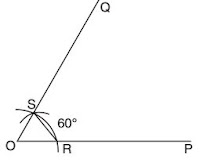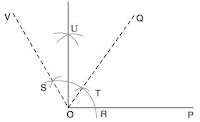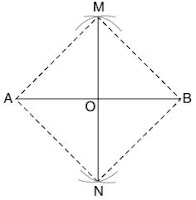>

## Revision Notes of Chapter 11 Construction Class 9th Math

Topics in the Chapter
• Basics of Constructions
• Construction of Angle Bisector
• Construction of Important Angles with some measurement.
• Construction of Perpendicular Bisector
• Construction of a triangle, given its base, difference of the other two sides and one base angle.
• Construction of a triangle of given perimeter and base angles.
Basics of Constructions
• A geometrical construction means to draw geometrical figures, such as an angle, a circle, a triangle, a quadrilateral, and a polygon, etc.

• We normally use all or some of the following instruments for drawing geometrical figures:
(i) A protractor
(ii) A pair of compasses
(iii) A pair of set squares
(iv) A pair of dividers

Construction of Angle Bisector

1. Steps for construction of Angle Bisector for a given angle.

Given: ∠POQ.
Construction: To construct the bisector of ∠POQ.
Steps of Construction:1. With O as centre and any suitable radius, draw  an arc to meet OP at R and OQ at S.
2. With R as centre and any suitable radius (not  necessarily) equal to radius of step 1 (but > 1/2 RS),  draw an arc. Also, with S as centre and same  radius, draw another arc to meet the previous arc  at T.
3. Join OT and produce it, then OT is the required  bisector of ∠POQ.

2. Construction of Important Angles with some measurement.

(i) To construct an angle of 60°

Steps of Construction:1. Draw any line OP.
2. With O as centre and any suitable radius, draw  an arc to meet OP at R.
3. With R as centre and same radius (as in step 2),  draw an arc to meet the previous arc at S.
4. Join OS and produce it to Q, then ∠POQ = 60°.

(ii) To construct an angle of 30°

Steps of Construction:1. Construct ∠POQ = 60° (as above).
2. Bisect ∠POQ (as in construction I). Let OT be the bisector of ∠POQ, then ∠POT = 30°.

(iii) To construct an angle of 120°

Steps of Construction:1. Draw any line segment OP.
2. With O as centre and any suitable radius, draw  an arc to meet OP at R.
3. With R as centre and same radius (as in step 2), draw an arc to meet the previous arc at T. With T as  centre and same radius, draw another arc to cut  the first arc at S.
4. Join OS and produce it to Q, then ∠POQ = 120°

(iv) To construct an angle of 90°

Steps of Construction:1. Construct ∠POQ = 60°.
2. Construct ∠POV = 120°.
3. Bisect ∠QOV.
4. Let OU be the bisector of ∠QOV, then ∠POU = 90°.

(v) To construct an angle of 45°

Steps of Construction:1. Construct ∠AOP = 90°
2. Bisect ∠AOP.
3. Let OQ be the bisector of ∠AOP, then ∠AOQ = 45°.

3. Construction of Perpendicular Bisector

Given: Any line segment AB.
Construction: To construct a perpendicular bisector of line segment AB.

Steps of Construction:1. Draw a line segment AB.
2. Taking A and B as the centres and radius of more than half the length of AB, draw arcs on both  sides of AB.
3. Let these arcs intersect each other at points M and N.  4. Join the points of intersection M and N. Thus, MN is the required perpendicular bisector of AB.

4. Construction of a triangle, given its base, difference of the other two sides and one base angle.

Construction: Construct a triangle with base of length 7·5 cm, the difference of the other two sides is 2·5 cm, and  one base angle of 45°.
Given: In DABC, base BC = 7·5 cm, the difference of the other two  sides, AB – AC or AC – AB = 2·5 cm and one base angle is 45°.
Required: To construct the DABC.

Case (i) AB – AC = 2·5 cm.Steps of Construction:
1. Draw BC = 7·5 cm.
2. At B, construct ∠CBX = 45°.
3. From BX, cut off BD = 2·5 cm.
4. Join CD.
5. Draw the perpendicular bisector RS of CD intersecting  BX at a point A.
6. Join AC. Then DABC is the required triangle.

Case (ii) AC – AB = 2·5 cm.Steps of Construction:

1. Draw BC = 7·5 cm.
2. At B, construct ∠CBX = 45° and produce XB to  form a line XBX’.
3. From BX’, cut off BD’ = 2·5 cm.
4. Join CD’.
5. Draw the perpendicular bisector RS of CD’  intersecting BX at a point A.
6. Join AC. Then DABC is the required triangle.

5. Construction of a triangle of given perimeter and base angles.

Construction: Construct a triangle with perimeter  11·8 cm and base angles 60° and 45°.
Given: In DABC, AB + BC + CA = 11·8 cm, ∠B = 60° and ∠C = 45°.
Construction: To construct the DABC.Steps of Construction:
1. Draw DE = 11·8 cm.
2. At D, construct ∠EDP = 1/2 of 60° = 30° and at E, construct ∠DEQ = 1/2 of 45° = 22½°.
3. Let DP and EQ meet at A.  4. Draw a perpendicular bisector of AD to meet DE at B.
4. Draw a perpendicular bisector of AE to meet DE at C.
5. Join AB and AC. Then DABC is the required triangle.